output.to from Sideway

Draft for Information Only

# Content

```  Sound Wavefronts    Plane waves    Cylindrical Waves    Spherical Waves```

## Sound Wavefronts

### Plane waves

Plane waves are the form of wave propagation in one dimension. The wavefronts of plane waves are planes perpendicular to the propagation direction.

Plane waves are generated by a plane source. The surface area of wavefront does not vary with the distance away from the sound source. Since there is no geometrical divergence along the propagation path, both the sound intensity and sound pressure remain unchanged.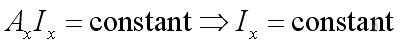and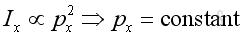where

Ax is the surface area at distance x and is a constant
Ix is the sound intensity at distance x
px
is the sound pressure at distance x

Therefore, both the sound intensity level and the sound pressure level remains constant with distance away from the source.

Plane waves propagation can be found in musical pipe instrument, e.g. flutes, clarinets, or noise in ducts.

### Cylindrical Waves

Cylindrical waves are the form of wave propagation in two dimension. The wavefronts of cylindrical waves are coaxial cylinders with axis of symmetry normal to the propagation direction.

Cylindrical waves are generated by a line source. The surface area of wavefront increases with the distance away from the sound source. Since there is geometrical divergence along the propagaton path, both the sound intensity and sound pressure  decreases with distance away from the source.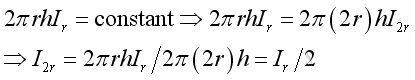and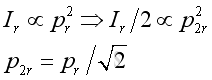where

2πrh is the surface area at distance r
Ir is the sound intensity at distance r
pr is the sound intensity at distance r
2π(2r)h is the surface area at distance 2r
I2r is the sound intensity at distance 2r
p2r is the sound intensity at distance 2r

Therefore, both the sound intensity level in term of I and sound pressure level in term of p2  decrease by 3dB as the distance of receiver away from the source is increased by double.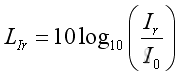and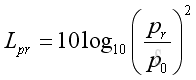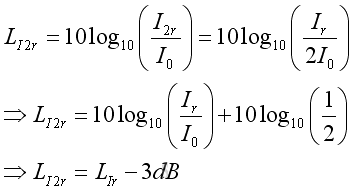and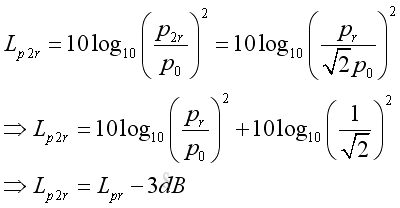where

LIr is the sound intensity level at distance r
Lp2r  is the sound pressure level at distance r
Ir is the sound intensity at distance r
Io is the sound intensity reference
pr is the sound intensity at distance r
po is the sound pressure reference
LI2r  is the sound intensity level at distance 2r
Lp2r  is the sound pressure level at distance 2r
I2r is the sound intensity at distance 2r
p2r is the sound intensity at distance 2r

Cylindrical waves propagation can be found in sound radiation from a circular pipes or duct, or noise field in an open space radiated by a lane of closely spaced vehicles.

### Spherical Waves

Spherical waves are the form of wave propagation in three dimension. The wavefronts of spherical waves are concentric spheres with center at the center of propagation in all directions.

Spherical waves are generated by a point source. The surface area of wavefront increases with the distance away from the sound source. Since there is geometrical divergence along the propagation path, both the sound intensity and sound pressure level decreases with distance away from the source.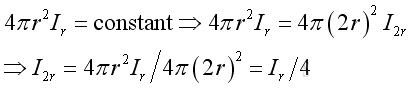and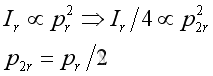where

4πr2 is the surface area at distance r
Ir is the sound intensity at distance r
pr is the sound intensity at distance r
4π(2r)2 is the surface area at distance 2r
I2r is the sound intensity at distance 2r
p2r is the sound intensity at distance 2r

Therefore, both the sound intensity level in term of I and sound pressure level in term of p2 decrease by 6dB as the distance of receiver away from the source is increased by double.and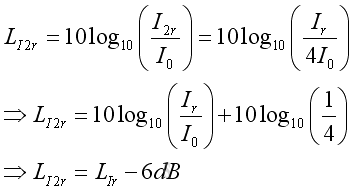and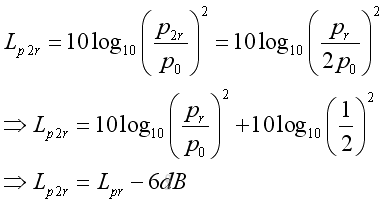where

LIr is the sound intensity level at distance r
Lp2r  is the sound pressure level at distance r
Ir is the sound intensity at distance r
Io is the sound intensity reference
pr is the sound intensity at distance r
po is the sound pressure reference
LI2r  is the sound intensity level at distance 2r
Lp2r  is the sound pressure level at distance 2r
I2r is the sound intensity at distance 2r
p2r is the sound intensity at distance 2r

Spherical waves propagation in practical can be found by approximating a sound source with source dimension and radiated wavelength much smaller than the distance away from the receiver.

©sidewayReferences

1. Michael P. Norton; Denis G. Karczub,, 2003, Fundamentals of Noise and Vibration Analysis for Engieer, Cambridge, United Kingdom
2. G. Porges, 1977, Applied Acoustics, Edward Arnold Limited, Britain
3. Conrad J. Hemond, 1983, Engineering Acoustics & Noise Control, Prentice-Hall, USA
4. F. Fahy, 2001, Foundations of Engineering Acoustics, Academic Press, UKID: 100900010 Last Updated: 9/8/2010 Revision: 0 Ref:Home (5)

Business

Management

HBR (3)

Information

Recreation

Hobbies (7)

Culture

Chinese (1097)

English (336)

Reference (66)

Computer

Hardware (149)

Software

Application (187)

Digitization (24)

Numeric (19)

Programming

Web (644)CSS (SC)

ASP.NET (SC)

HTML

Knowledge Base

Common Color (SC)

Html 401 Special (SC)

OS (389)MS Windows

Windows10 (SC)

.NET Framework (SC)

DeskTop (7)

Knowledge

Mathematics

Formulas (8)

Number Theory (206)

Algebra (20)

Trigonometry (18)

Geometry (18)

Calculus (67)

Complex Analysis (21)

Engineering

Tables (8)

Mechanical

Mechanics (1)

Rigid Bodies

Statics (92)

Dynamics (37)

Fluid (5)

Control

Acoustics (19)

Biology (1)

Geography (1)

Latest Updated Links

Copyright © 2000-2019 Sideway . All rights reserved Disclaimers last modified on 10 Feb 2019